HOW TO

# Determine how much of a route is traversed along edges with specific attribute values

## Summary

Instructions provided describe how to determine how much of a route is traversed along edges with specific attribute values.

Cost accumulation can be used to extract cost values for edges that exhibit specific attribute values. It can be used to answer these types of questions:

• How much distance of the route is traversed on two-lane roads? Highways?

• How much time is spent on an interstate? Local roads?

## Procedure

Provided that the desired information exists as attribute values, Network Analyst can calculate accumulation values for them based on cost. The workflow is to create attributes, assign evaluators, and set accumulation.

1. Go the Network Dataset Properties > Attributes tab.
`Network Dataset Properties Window`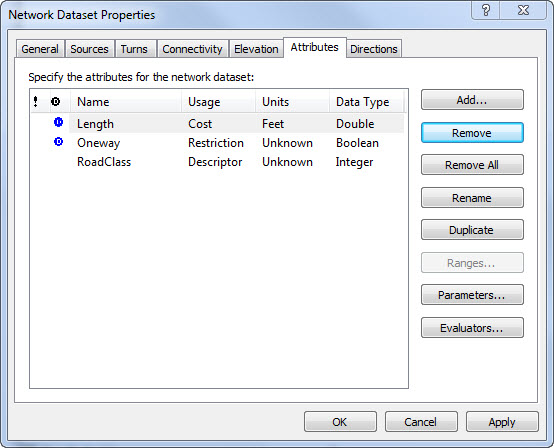2. Click Add and create a new cost attribute with a name that reflects the type of accumulation desired.
`Costs can be distance or time based`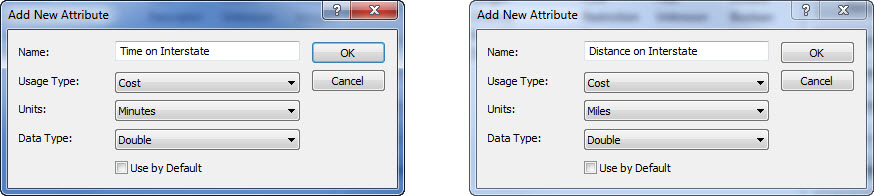3. Click Evaluators and set the type to Field for both directions. Then click the Evaluator Properties button on the right to open the Field Evaluators window.
`Evaluators are used to specify the values for attributes`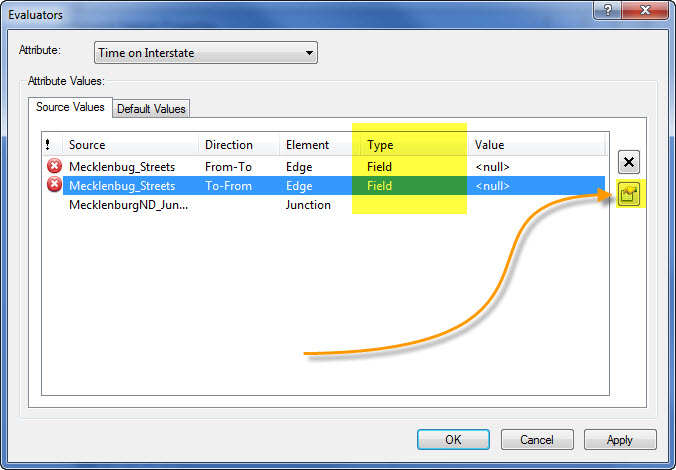4. Enter an If statement that calculates the Time or Distance value if certain conditions are met. In the example, as long as the road has an ACC type of 1 (interstates) then length of time or distance to traverse is calculated. Otherwise, nothing is calculated (0). The length of time or distance to traverse may already be in field format in the table, or it can be calculated on the fly as in the example:

Time-based:

`Code:If [ACC] = "1" Then x = ([Shape_Length]/5280) / [SPEED])Else x = 0End If`

Distance-based:

`Code:If [ACC] = "1" Then x = [Shape_Length]/5280Else x = 0End If`

`The VB Script varies for time and distance costs`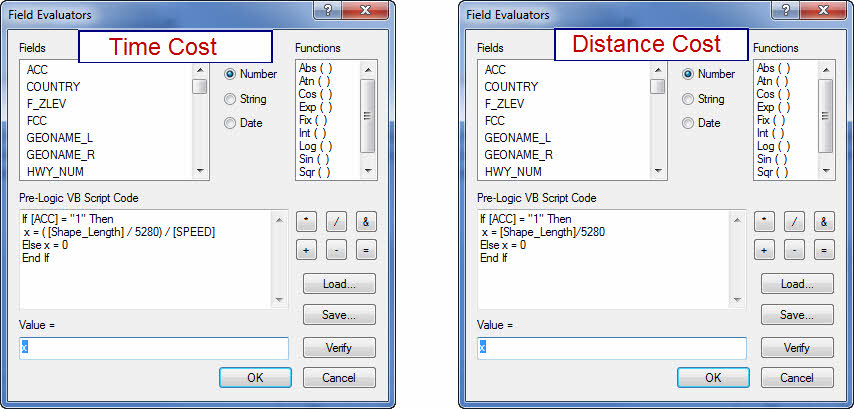5. Copy the Pre-Logic formula and click OK. Click evaluator properties for the other direction and paste the Pre-Logic and value formula here. Click OK and OK in the Network Dataset Properties dialog.
6. Rebuild the network (right-click the Network Dataset in ArcCatalog > Build or the Build Network Dataset button on the Network Analyst toolbar).
7. Add the network dataset to a map and create a new route using the Network Analyst toolbar. Prior to solving the route, go to the route Properties > Accumulation tab and check the box next to the new attributes that were created.
`Attributes must be turned in the Accumulation tab`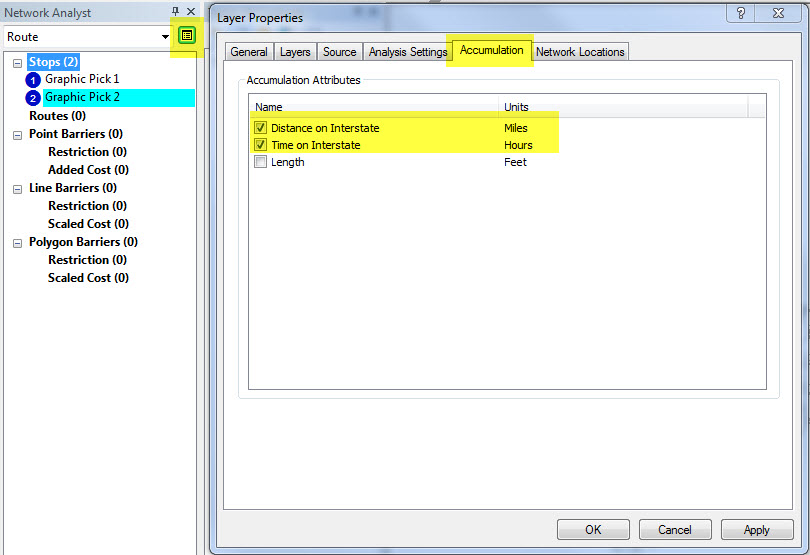8. Once the route is solved, it has the accumulation values for the new attributes. Additionally, if the routes are exported to a feature class/shapefile, it also includes the accumulation values.
`Results`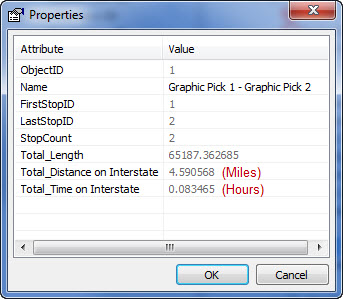Article ID:000011563

Software:
• ArcMap

Get help from ArcGIS experts

Contact technical support